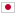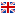Contest Duration: - (local time) (180 minutes) Back to Home
E - Game/Time Limit: 2 sec / Memory Limit: 256 MB

### Problem

There is a video game. In the game, there are N numbers of stages. There are 3 levels of difficulty and each stage has one of a difficulty in 1,2,3.

The number of stages of each difficulty is N_1,N_2,N_3 (N_1+N_2+N_3=N) For each difficulty, you know that the probability of completing the stage of that difficulty in one trial is P_1,P_2,P_3 (%).

You have to pay cost 1 to play the game. In one gameplay, you can play at least 1 stage, and at most 4 stages. The number of stages you can play with cost 1 depends as following.

• If you complete the first trial, you can continue to play the second trial. If you couldn't complete the first trial, that's the end of that play.
• If you complete the second trial, you can continue to play the final trial. If you couldn't complete the second trial, that's the end of that play.
• If you complete the final trial and also if the difficulty of that stage was 2 or 3, you can continue to play the extra trial. If not, that's the end of that play.

Before starting each first, second, final, and extra trial, you may choose any stage of any difficulty to play. Also, you may choose the stage you have already completed before.

For example, if you paid cost 1 and started the game, completed the first trial, and failed the second trial, that's the end of the play for that time. You can't play the final trial in that case.

Your aim is to complete all the N stages at least 1 time each. Regarding you followed a optimal strategy to minimize the total cost, calculate the expected value of cost you must pay to achieve that.

Whenever you choose the stage to play, you can choose any stage using the information about all stages you have tried including the stages you have completed at previous trial.

### Input

Input is given in the following format.

N_1 N_2 N_3
P_1 P_2 P_3

• On the first line, you will be given three integers N_1,N_2,N_3 (0 \leq N_1,N_2,N_3 \leq 100), the number of stages of each difficulty separated by space, respectively.
• On the second line, you will be given three integers P_1,P_2,P_3 (1 \leq P_1,P_2,P_3 \leq 100), the probability of completing the stage of each difficulty in percentage separated by space, respectively.

### Output

Output one line containing the expected value of cost you must pay to complete all stages when you followed the optimal strategy to minimize the total cost. Your answer is considered correct if it has an absolute or relative error less than 10^{-7}. Make sure to insert a line break at the end of the line.

### Input Example 1

3 0 1
100 100 100


### Output Example 1

1.0


There are 4 stages. you can surely complete the stage of any difficulty at once.

Pay cost 1 to start the gameplay. For each trial, choose the stage as following and you can complete all the stages with no additional cost.

• For the first trial, choose the stage of difficulty 1.
• For the second trial, choose the stage of difficulty 1.
• For the final trial, choose the stage of difficulty 3.
• For the extra trial, choose the stage of difficulty 1.

### Input Example 2

100 100 100
100 100 100


### Output Example 2

75.0


### Input Example 3

3 0 1
50 100 50


### Output Example 3

5.0


### Input Example 4

4 1 1
50 25 10


### Output Example 4

17.01875


### Input Example 5

11 13 17
75 50 25


### Output Example 5

69.106100438